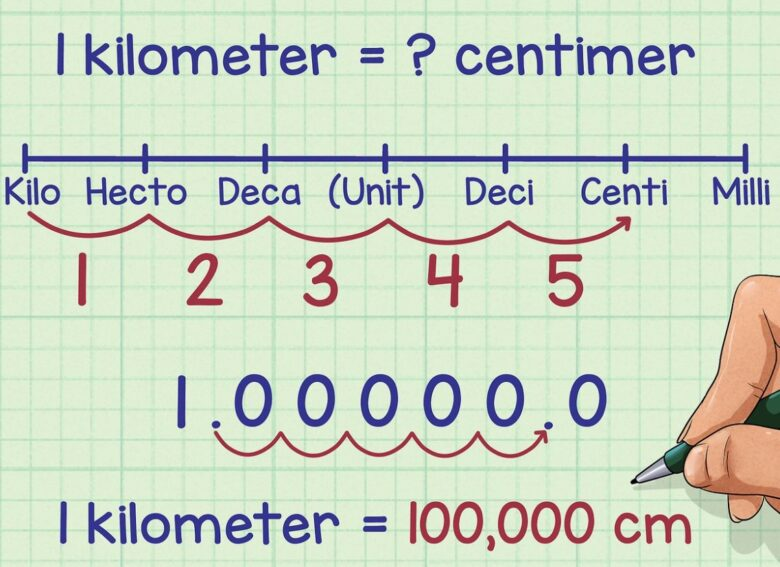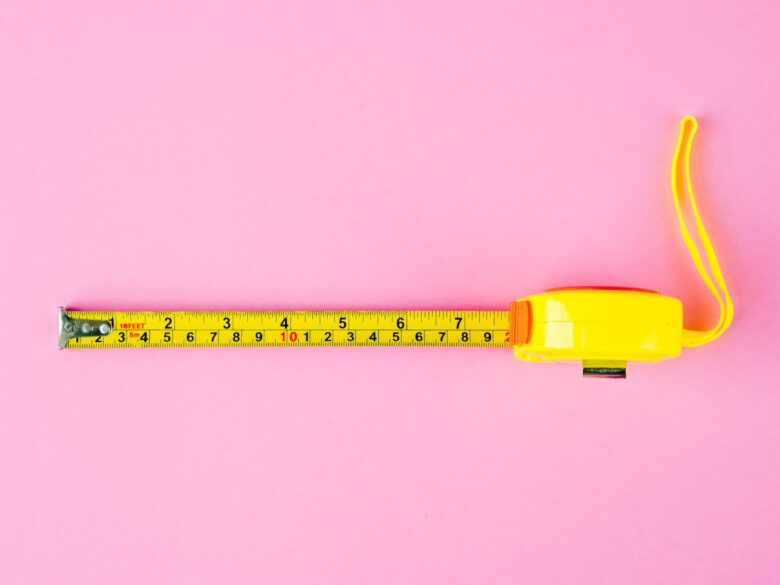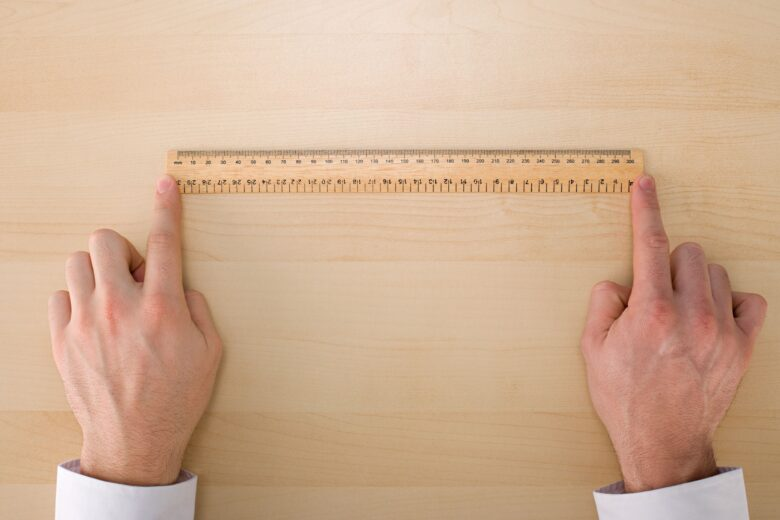Home Tips 6 Tips for Understanding Metric System Units and Conversions

# 6 Tips for Understanding Metric System Units and Conversions

The metric system is a decimal-based system of measurement that is used in many countries around the world. In fact, all but three countries in the world officially use the metric system as their official system of measurement. The only countries that don’t are USA, Lybia and Myanmar.

The system is based on the meter and liter and uses prefixes to denote different measurements. The metric system can be used to measure weight, distance, volume, and temperature.

There are many online tools and resources available to help you understand the metric system better, as well as opportunities to learn more about it through formal education or training programs. Familiarizing yourself with the basics of the metric system will help make conversions between units easier and faster.

However, if you don’t know where to start, this process can be a bit tricky. Therefore, we’ve decided to help you out. If that sounds interesting, take a look at our six tips, and we promise you that you’ll grasp the metric system rather easily.

## 1. Familiarize Yourself With The Metric System PrefixesSource: wikihow.com

The first step to getting familiar with the metric system is to understand its prefixes. As we’ve said, the metric system is based on the meter and liter and uses prefixes to denote different measurements. The most common prefixes are:

• Kilo = 1,000
• Hecto = 100
• Deka = 10
• Deci = 0.1
• Centi = 0.01
• Milli = 0.001
• Micro = 0.000001

And so on.

Of course, we could go up and down for pretty much infinity, but as we get smaller and smaller as well as bigger and bigger, we get into exponents, but we’ll save that math lesson for another time.

## 2. Understand The Base Unit For Each Metric System Measurement And How It Is DerivedSource: rd.com

Now that you are more familiar with metric system prefixes, it’s time to focus on the base units of measurement. The most common base units in the metric system are:

• Meter (m) – The meter is the metric unit of length and is equal to 3.28 feet – for our American friends.
• Gram (g) – The gram is the metric unit of mass and one-thousandth of a kilogram. It is also equal to 0.035 ounces.
• Liter (l) – The liter is the metric unit of volume, usually used to measure liquids. One liter in ounces is about 33.81 fluid ounces.
• The Kelvin (K) – The Kelvin is the metric unit of temperature. However, it is very rarely used to measure temperature. Instead, countries using the metric system are using Celsius. One Celsius equals 273.15 Kelvin or 33.8 Fahrenheit.
• Ampere (A) – The ampere is the metric unit of electric current.

## 3. Practice Converting Between Metric System Units

Now that you know about the base units in the metric system, it’s time to learn about some common conversions. Here are some of the most common conversions in the metric system:

• 1 meter = 100 centimeters
• 1 meter = 1000 millimeters
• 1 kilometer = 1000 meters
• 1 liter = 1000 milliliters
• 1 kilogram = 1000 grams

These are just a few of the most common metric system conversions. As you can see, many of these conversions are simply moving the decimal point over by a certain number of places. This is why it’s so important to memorize the prefixes associated with each unit – it will make conversion much simpler.

If you need to convert between two units that aren’t listed here, there are plenty of resources like https://becalculator.com/ to help you, but before you turn to online tools, we’d suggest you practice on your own.

## 4. Memorize Common Conversions Between The Metric System And Imperial SystemSource: student-tutor.com

Now that you know the basics of the metric system, it’s time to start memorizing some common conversions between the metric and imperial systems.

This will help make conversions quicker and easier, and eventually, you’ll be able to do them without even thinking about it. Here are some of the most common conversions to start with:

• 1 inch = 2.54 cm
• 1 foot = 30.48 cm
• 1 meter = 3.28084 feet
• 1 Celsius = 33.8 Fahrenheit
• 1 yard = 91.44 cm
• 1 liter = 33.814 fluid ounces
• 1 kilogram = 2.20 pounds

Of course, there are many other conversions not listed here, but these are some of the most common ones that you’re likely to come across. We suggest memorizing a few main ones, like the ones we mentioned just now, and if you end up needing to learn more – you can.

## 5. Understand How To Use A Conversion Factor To Convert Between The Metric System UnitsSource: thoughtco.com

Now that you know about some common conversions in the metric system, it’s time to learn how to use a conversion factor. A conversion factor is simply a ratio between two units of measurement that can be used to convert between them.

For example, let’s say we want to convert from centimeters to meters. We know that there are 100 centimeters in 1 meter, so our conversion factor would be 100:1.

To use the conversion factor, we simply need to multiply the number of centimeters by the conversion factor. So, if we wanted to convert 150 centimeters to meters, we would multiply 150 by the conversion factor of 100:1. This would give us the answer we’re looking for.

## 6. Practice Using The Metric System In Everyday Life To Become More Comfortable With ItSource: sugarandsparrow.com

Now that you know the basics of the metric system, it’s time to start using it in your everyday life. Here are a few ways you can do this:

• Whenever possible, use metric units when shopping. For example, buy milk by the liter instead of by the gallon, and buy your fruit and vegetables by the kilogram instead of by the pound.
• When cooking, use metric measurements instead of imperial. For example, use milliliters or liters when measuring liquids and grams or kilograms when measuring solids.
• Use metric units when talking about temperature.